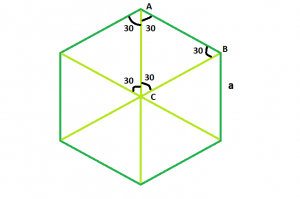GeeksforGeeks App
Open AppBrowser
Continue

# Find length of Diagonal of Hexagon

Given a regular hexagon of side length a, the task is to find the length of it’s diagonal.
Examples

```Input : a = 4
Output : 8

Input : a = 7
Output : 14```From the diagram, it is clear that the triangle ABC is an equilateral triangle, so
AB = AC = BC = a.
also it is obvious, diagonal = 2*AC or 2*BC
So the length of diagonal of the hexagon = 2*a
Below is the implementation of the above approach:

## C++

 `// C++ Program to find the diagonal``// of the hexagon``#include ``using` `namespace` `std;` `// Function to find the diagonal``// of the hexagon``float` `hexadiagonal(``float` `a)``{``    ``// side cannot be negative``    ``if` `(a < 0)``        ``return` `-1;` `    ``// diagonal of the hexagon``    ``return` `2 * a;``}` `// Driver code``int` `main()``{``    ``float` `a = 4;` `    ``cout << hexadiagonal(a) << endl;` `    ``return` `0;``}`

## C

 `// C Program to find the diagonal``// of the hexagon``#include ` `// Function to find the diagonal``// of the hexagon``float` `hexadiagonal(``float` `a)``{``    ``// side cannot be negative``    ``if` `(a < 0)``        ``return` `-1;`` ` `    ``// diagonal of the hexagon``    ``return` `2 * a;``}`` ` `// Driver code``int` `main()``{``    ``float` `a = 4;``    ``printf``(``"%f\n"``,hexadiagonal(a));``    ``return` `0;``}`

## Java

 `// Java Program to find the diagonal``// of the hexagon` `import` `java.io.*;` `class` `GFG {` `// Function to find the diagonal``// of the hexagon``static` `float` `hexadiagonal(``float` `a)``{``    ``// side cannot be negative``    ``if` `(a < ``0``)``        ``return` `-``1``;` `    ``// diagonal of the hexagon``    ``return` `2` `* a;``}` `// Driver code`  `    ``public` `static` `void` `main (String[] args) {``            ``float` `a = ``4``;` `    ``System.out.print( hexadiagonal(a));``}``}` `// This code is contributed``// by shs`

## Python3

 `# Python3 Program to find the diagonal``# of the hexagon` `# Function to find the diagonal``# of the hexagon``def` `hexadiagonal(a):` `    ``# side cannot be negative``    ``if` `(a < ``0``):``        ``return` `-``1` `    ``# diagonal of the hexagon``    ``return` `2` `*` `a`  `# Driver code``if` `__name__``=``=``'__main__'``:``    ``a ``=` `4``    ``print``(hexadiagonal(a))` `# This code is contributed by``# Shivi_Aggarwal`

## C#

 `// C# Program to find the diagonal``// of the hexagon``using` `System;` `class` `GFG {` `    ``// Function to find the diagonal``    ``// of the hexagon``    ``static` `float` `hexadiagonal(``float` `a)``    ``{``        ``// side cannot be negative``        ``if` `(a < 0)``            ``return` `-1;``        ` `        ``// diagonal of the hexagon``        ``return` `2 * a;``    ``}` `// Driver code``public` `static` `void` `Main()``{``   ``float` `a = 4;``   ``Console.WriteLine( hexadiagonal(a));``}``}` `// This code is contributed``// by anuj_67..`

## PHP

 ``

## Javascript

 ``

Output:

`8`

Time complexity: O(1), since there is no loop or recursion.

Auxiliary Space: O(1), since no extra space has been taken.

My Personal Notes arrow_drop_up